## Introduction

There seems to be an inevitability to the notion of anti-gravity. Given how effectively other systems of nature can be exercised, such as electromagnetics, chemical interactions, thermodynamics, sound, and so on; gravitation can seem an odd holdout. Why can’t we control gravity like we can electricity? Looking into gravitation and understanding its nature in terms of General Relativity brings quick clarity to the question, and reveals the difficulty of the problem.

If we start with the simple goal of either directly manipulating gravity or finding a way of directly overcoming it, then the first and most obvious step is to understand how it occurs naturally. General Relativity tells us that gravity is a consequence of the curvature of spacetime. Ignoring questions of Dark Matter for the moment, this curvature is caused by the presence of mass and/or energy, which distorts spacetime into Gravity Wells and then becomes enveloped within them.

This rather straightforward understanding tells us that if manipulation of gravity is possible at all, the only lever at our disposal for doing so is matter itself. If we assume for this exercise, that our understanding of elementary particles and their interactions, as described by the Standard Model of Particle Physics, is at least, mostly complete, and that anti-gravity is indeed, ultimately achievable, then the search for it reduces to finding the base mechanisms, the key interactions within that model that can be exercised to artificially modify spacetime curvature or interfere with the way matter interacts with it.

The concepts that follow are expressed in terms of the Standard Model of Particle Physics. They spell out, at a high-level, the properties, behaviors and interactions that can be orchestrated to modify a gravitational field.

## Mass

The image below is a loose depiction of gravitation. The grid represents spacetime, and the distorted appearance in the area immediately surrounding the spheres are gravity wells. In the absence of matter, spacetime tends to be flat. There are non-trivial exceptions, but we can safely make that assumption for purposes at hand. With respect to the image, if the spheres were not present, the assumption is that the grid would be uniform.

The depressions surrounding the two spheres suggest that if they were to come into range where their depressions began to appreciably overlap, that they would accelerate towards one another - they would begin to fall into the expanding depression created from the merging of their individual depressions.

For illustrative purposes, we must now level-set using an unpopular and somewhat flawed analogy. We’ll use it to set a baseline, and then move on.

If we assume for a moment that we have benefit of an artificially perfect trampoline, we could reproduce the analogy depicted in the image marginally well. If nothing were on it, it would be flat. If we then imagined a heavy bowling ball and a large ball of Styrofoam, we could use them to experiment with various principles of gravitation in a rather crude, but not entirely invalid way.

The diameter of a Styrofoam ball with the same mass as a heavy bowling ball would be much greater due to its lower density. If we placed them onto the trampoline one at a time, they would sink to different depths. The bowling ball is smaller, so it will sink further despite the fact that it weighs no more than the Styrofoam because its mass is denser, more focused. Density is equal to mass divided by volume.

Looking at the trampoline edge-on, the slope of the well caused by the bowling ball would be steeper at the center, whereas the larger footprint of the Styrofoam ball would result in a broader depression that reached closer to the edges of the trampoline. Interestingly, edge-on, the area of the depression caused by each of the two would be about the same. This gives us an imperfect clue about visualizing mass, density and weight with respect to gravity. The mass of the two objects are the same, but the density is different, and therefore their impact on the underlying trampoline is different as shown in the exaggerated figure below.Looking edgewise at the trampoline, the length of an imaginary line between the deepest point of the trampoline beneath the ball, and the point where the surface of the trampoline would be if there were nothing on it at all, is analogous to the strength of the pull of gravity. From this point, it’s not difficult to imagine what might happen if the bowling ball were to arbitrarily change size. If its mass were to stay the same, but its diameter increased to the size of the Styrofoam, then the depth to which it sank into the surface of the trampoline would decrease correspondingly, meaning the ball would rise.

Unfortunately, with respect to real gravity, the analogy doesn’t translate well. A given quantity of mass has a predictable and measurable gravitational attraction to Earth. Although the ball may rise on a trampoline, it would not rise into the air as a result of decreasing its density. Here on Earth, the Styrofoam and the bowling ball are equally attracted to the earth, meaning they weigh the same (ignoring minute differences due to the distributions of their mass). Of course, if the ball grew to a size where its density became less than the surrounding air, it would rise, but only because the denser air surrounding it would push it upward, which would have nothing to do with modification of the surrounding gravitational field. To derive anything meaningful from the analogy, we must shift perspectives.

The Earth’s gravitational field is rigid. All of the matter that makes up the Earth interacts with spacetime, and in aggregate, produces the gravity well that keeps our feet planted to its surface. It seems unlikely that any lever exists that could fundamentally alter the Earth’s gravity. If we accept this premise, then we must look elsewhere for a solution.

## Standard Model of Particle Physics

Physicists have long-since identified the four fundamental interactions of matter; that is, the forces that matter reacts to (ignoring the Higgs Field for now). That tells us that if we hope to overcome gravity, we already know what the levers are, we must only discover how to operate them.

One of the basic interactions is gravitation itself, but since gravitation is the interaction we’re attempting to manipulate, we must set it aside. Doing so takes us a step further. It means that we have reduced our range of possibilities. If we want to impact a gravitational field, we must do it via one or more of the remaining three. So what are they? The Electromagnetic Force, the Strong Nuclear Force and the Weak Nuclear Force.

In nature, the weak force plays a role in nuclear decay and in the types of physics that underlie the inner workings of the sun. For the most part, its usefulness appears to be confined to those roles. It has no apparent role to play in the manipulation of gravity, so we can set it aside as well.

This leaves us with Electromagnetics and the Strong Nuclear Force. The final solution involves using these two forces in concert; leveraging electromagnetics to indirectly influence the strong force, which then triggers behaviors that impact the surrounding gravitational field.

## The Strong Nuclear Force

One of the primary functions of the strong force is to bind the nucleus of atoms together. A nucleus is made up of a combination of protons, which are positively charged particles, and neutrons, which have no charge. The catch is, in electromagnetics, opposites attract. A positively charged particle is attracted to a negative one, and vice versa; but two positively charged particles repel one another. Same with negatively charged ones. This means that the only way the nucleus of an atom stays intact is if something prevents all the positively charged particles within it from separating. That’s what the strong force does. The positive particles within the nucleus are always trying to push away from one another, but the strong force binds them together, preventing it. Because the strong force is 137 times stronger than the repulsive force of the charged protons, the nucleon and the atom remain intact.

There are two kinds of nucleons; protons and neutrons. These are the particles that make up the nucleus of atoms. The common understanding is that protons have a positive charge, while neutrons have no charge. As a net value, that is correct. But, if you were to look inside of them, you would find that they are made up of smaller, subnuclear particles, called quarks, which do have charge.

All nucleons are comprised of three quarks. In simple terms, there are two types; up quarks and down quarks. There are variant types, but they can be ignored for our purposes. Up quarks have an elementary positive charge of , whereas down quarks have a negative charge of ; the elementary charge being that of a single proton (e). Protons have two up quarks and one down quark, meaning that the net charge of all three quarks is one elementary charge; ⅔ + ⅔ - ⅓ = 1. Neutrons, on the other hand, have two down quarks and one up quark, with a net charge of zero; ⅔ -⅓ -⅓ = 0.

As mentioned above, without the strong nuclear force, the protons within atoms would spring away from each other, but the strong force also plays a role within nucleons. Just as protons would repel one another due to their positive charges, quarks with like charges would do the same. This means that even protons and neutrons would disband without the strong nuclear force to bind their internal parts together.

Interestingly, the strong force acts in a very specific way at sub-nucleon levels, and it involves yet another kind of charge, called the color charge. Unlike electromagnetics, which possesses two types of charge, being positive and negative, the color charge has three; red, green and blue. All quarks possess it. Note that the naming of this type of charge in terms of colors is only for convenience and reference, it has nothing whatever to do with typical color as we think of it. Simply think of each color as a label for a type of charge in the same way you would think of positive versus negative as labels for electrical charge. And, just as electrical charges of the same type repel one another, and opposites attract; like color charges also repel one another, but different colors attract. This means that a quark with a red charge will repel another quark with a red charge, but be attracted to a green or a blue one. Likewise, a green charge repels a green charge, but attracts a red or a blue.

The important thing to remember is that nucleons (protons and neutrons) contain three quarks, one of each color; red, green and blue. And, all quarks possess two types of charge; color and electromagnetic.

In a typical atom, nucleons are knotted together as tightly as possible because there is nothing to interfere with the pull of the strong force; that is, except for the comparatively feeble electromagnetic force. The diagram below provides a logical visualization of a grouping of three protons. The quarks within each proton are arranged such that their color charges align to form pairings with quarks of unlike color charges in neighboring protons. This works for neutrons too. The attraction between the quarks of unlike colors is the manifestation of the strong nuclear force within the nucleon. I’ve labelled this as, attractive pairing, in the diagram. Here, we see a red quark aligned with a green, a blue aligned with a red, and a blue aligned with a green.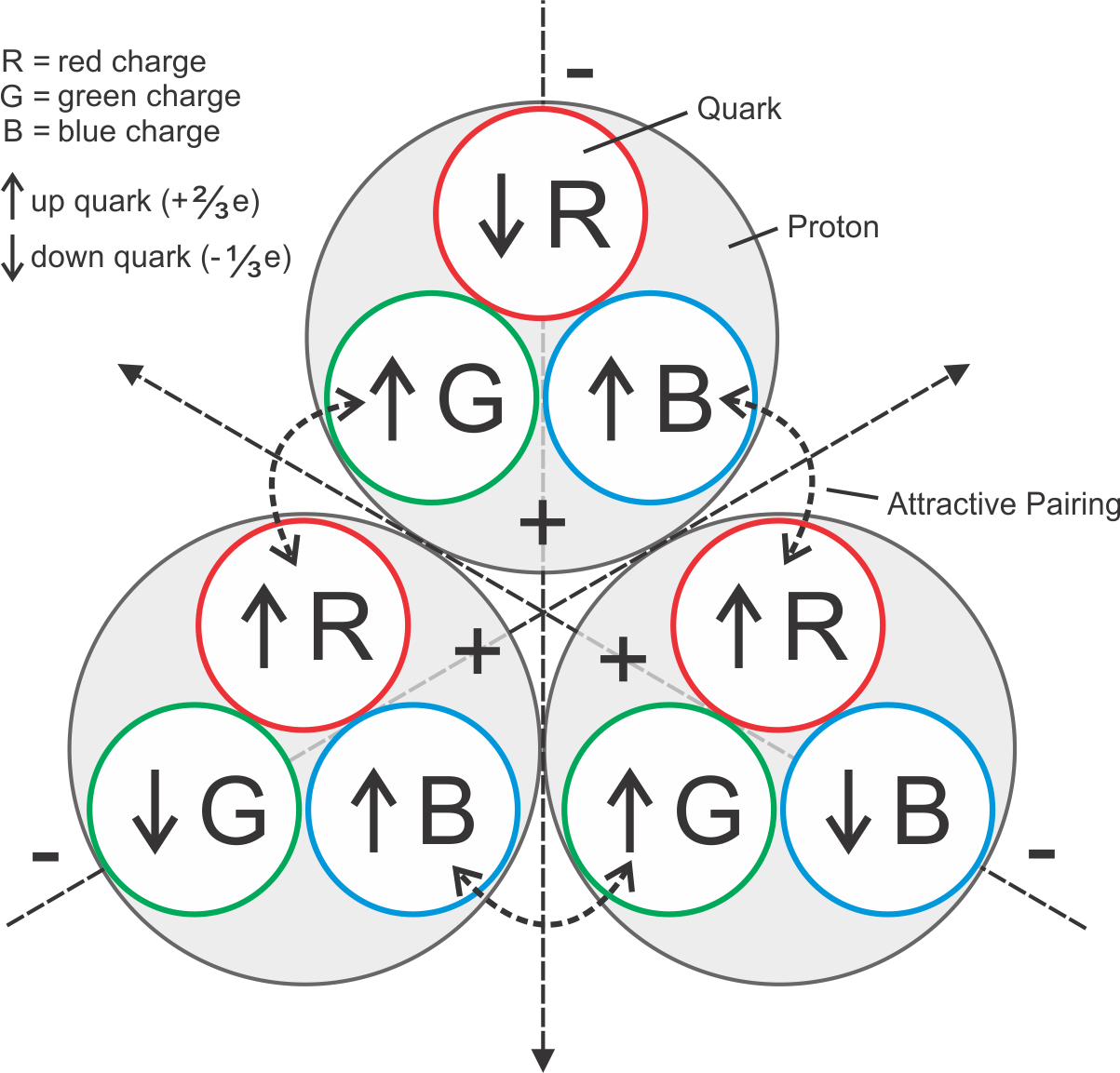Notice the charge symbols on the protons, which represent the aggregate electromagnetic charge based on the charge values of the individual quarks within them. The straight arrows passing through the protons depict the orientation of the electromagnetic charge. As you can see, the positively charged sides of the protons are facing one another due to the overriding power of the strong nuclear force (denoted with “+” symbols near the center of the diagram). This arrangement is precisely the reason that, in the absence of the strong force, the nucleons would disband.

Supposing for a moment that the protons could be aligned along the electromagnetic field as opposed to the color field, as is the natural state, it would turn the protons to a point where like color charges would begin to come into alignment. Consider the diagram below.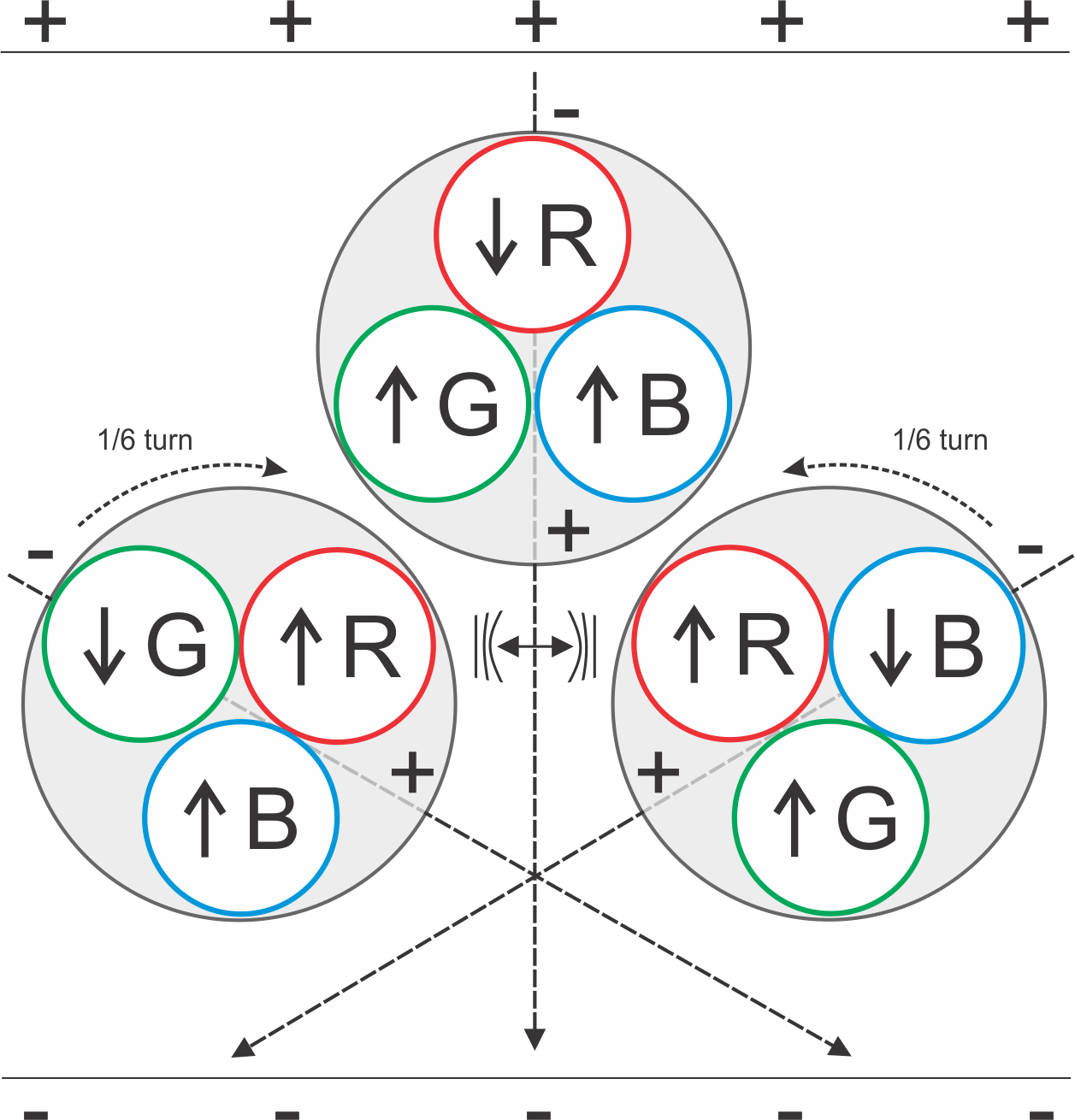Here we see that creating an electromagnetic field that is strong enough to influence the alignment of the protons by causing their orientations with respect to one another to shift by only 1/6th, begins to bring, in this logical example, the red quarks within the bottom two protons into alignment. As a result, they push apart. If the protons were brought into full electromagnetic alignment, the result could look like the following.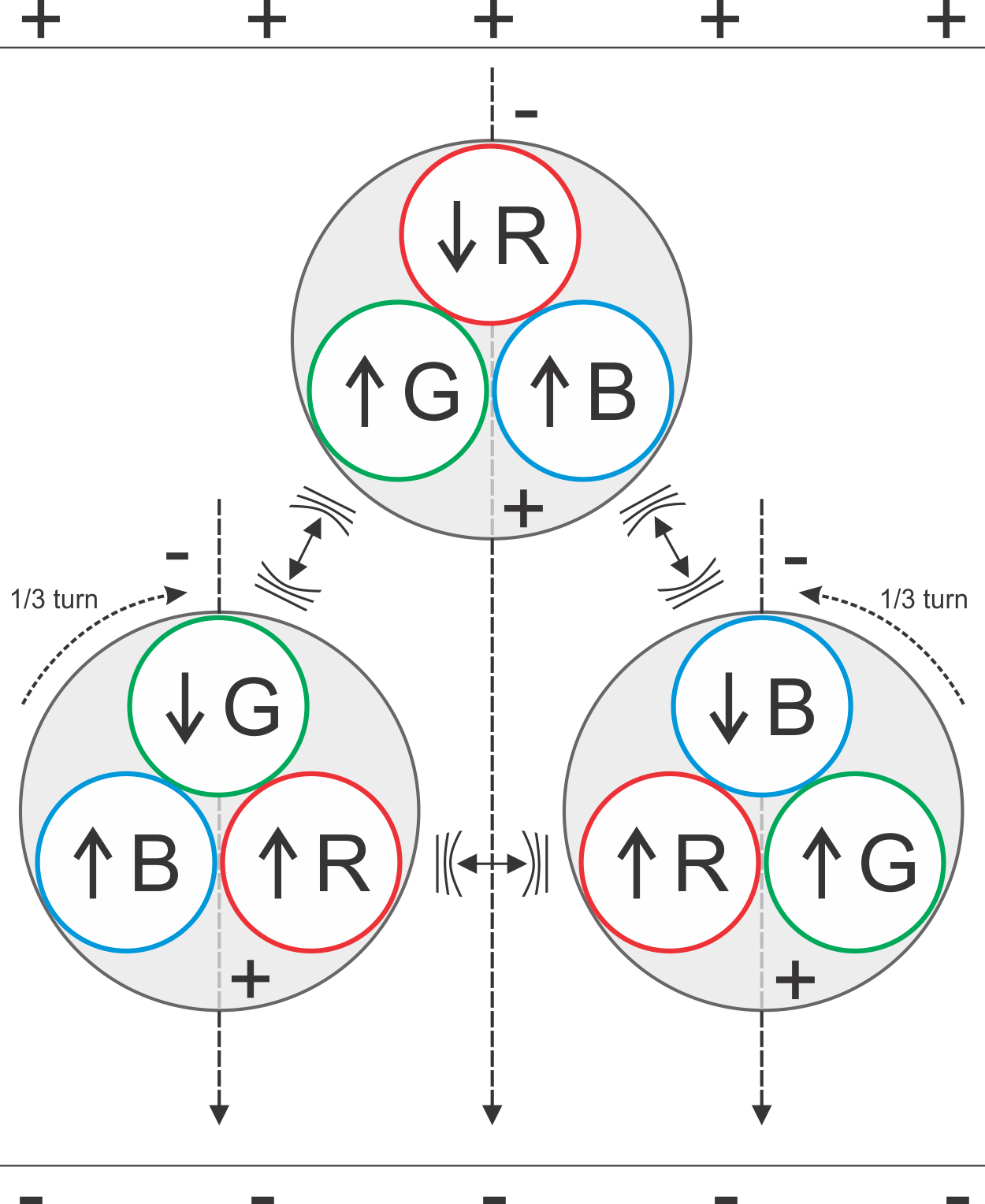Rotating the protons by only , positions their internal quarks from being paired with their unlike counterparts, to where the color charges are exactly aligned to achieve maximal repulsion. A reasonable conclusion is that, by interfering with the natural pairings of quarks according to color, and causing quarks with the same colors to face one another, that the protons would push apart. They would begin to separate.

This leads to a question: If this were possible, what would it accomplish? Would separating the protons have any impact on gravitation? Presumably, doing so would not change their mass. But, if we think about a light element such as helium with an atomic weight of ~4 as compared to copper with a weight of ~64, it could provide further insight. What makes one lighter than the other?

## Density

In the end, the viability of modifying a gravitational field as asserted in this document hinges entirely on density. Using electromagnetics to impact the strong nuclear interaction, and thereby, indirectly, the arrangement of nucleons within atoms, serves the singular purpose of modifying density. The question then becomes, does density matter?

The most obvious answer seems to be no. Protons and neutrons have a known weight, therefore, changing the density of any object comprised of them should have no practical bearing. Astronaut David Scott performed the famous feather and hammer gravity test on the moon during Apollo 15 as a convincing visual demonstration that mass and density have no measurable bearing on the attraction of gravity.

But there seems to be something of a contradiction here. Gravitation is an effect of the curvature of spacetime as caused by the presence of mass, and the extent of that curvature is a function of mass and density. We know, for example, that the gravitational force on Earth is much greater than on the moon or Mars due to differences in mass; but density plays just as large a role.

For illustrative purposes, consider the effect of distributing the matter that makes up Mars over a region of space the size of the Sun. The diameter of Mars is 4,200 miles, whereas the sun has a diameter of 864,000 miles. Given that, the comparatively small amount of matter contained within Mars would be so widely distributed that there would be no surface to land on and no appreciable gravity. There is a good chance that there would not be enough matter to create so much as a recognizable dust cloud. How could the same quantity of mass yield such wildly varying gravitational differences, all based on how it is arranged? The answer is density. The matter is too widely distributed to produce a gravity well of meaningful dimension. The individual particles would still have their own gravitational impacts, but they would be too widely distributed to produce a gravity field of appreciable consequence.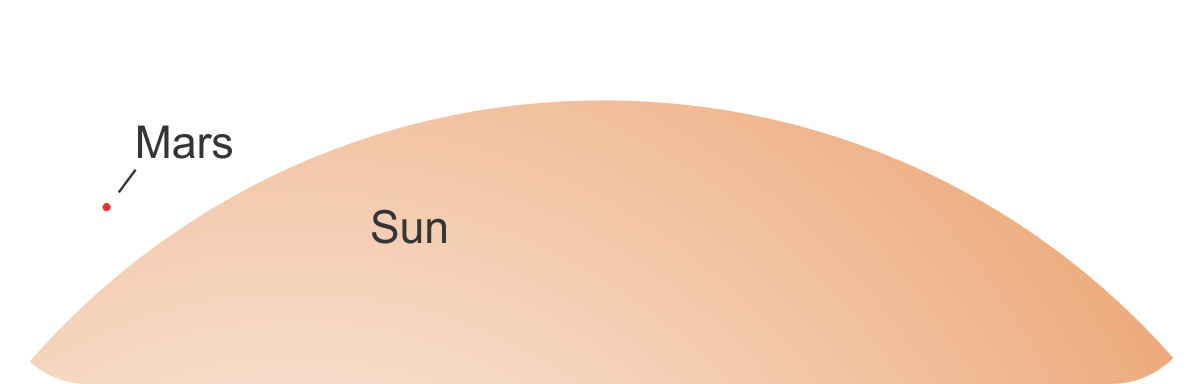Perhaps revisiting the trampoline analogy, which ended with unsatisfying results earlier in the paper, could yield further insight. There was an apparent disconnect between the way gravitation was depicted in the trampoline model and the way it plays out in the real world. According to the analogy, differences in the density of the bowling ball versus the Styrofoam ball had direct bearing on the gravitational attraction to the underlying source. Yet, this doesn’t happen in real life. Where is the disconnect? Where is the flaw in the model?

In fact, there are several. First, the trampoline isn’t representative of gravity at all. The surface of the trampoline isn’t pulling the objects into itself; instead, the assumption is that the entire trampoline is standing within a gravitational field. The bowling ball and the Styrofoam ball are pressed into the trampoline because it is positioned between them and, presumably, some large body which is the source of gravitation.

The second, more relevant issue is that the analogy treats each of the two balls as indivisible units. Meaning, the attraction of gravity plays out against each of the balls as if they were non-reducible objects. Real gravitation acts upon each individual particle within objects, not at the level of the objects that are constructed from them. In the analogy, each atom in the bowling ball presses down, not against the trampoline, but against the atoms between it and the trampoline. The aggregate downward force of all the vertically aligned atoms meets the trampoline surface at the bottom-most atom, which transfers the combined force of them all.

It can’t be depicted meaningfully in terms of the trampoline analogy, but if each individual atom that makes up the balls, somehow transferred its weight directly to the trampoline surface, the analogy would be at least, a step closer to having real-world physical applicability. The image above shows that the shape of an object has bearing on how it interacts with gravity, but because the impacts are vanishingly small, they are ignored for all practical purposes.

Another way to think of this is that each atom within the balls are subject to gravitational attraction to a measure entirely defined by their own mass and density. The incidental fact that they happen to be grouped together into balls has no appreciable bearing. Thinking of the balls as convenient groupings of the individual atoms within them, and understanding that each atom has its own weight, brings some clarity to the reasons a feather and a hammer appear to fall at the same rate if not interfered with by other forces, such as the resistance of air. Both the feather and the hammer are made up of atoms (granted, of different types), and each of those atoms has weight, which means they will fall individually or in groups, at a given rate.

Yet, we know that density is a factor. As matter coalesces in space, for example, it presses deeper and deeper into spacetime. What may begin as a very shallow gravity well can, nonetheless, cause nearby matter to slide into it, contributing its own mass. The impact is that the gravity well becomes deeper and stronger, extending its reach and attracting still more mass. This is the very type of process that leads to the formation of new stars and solar systems, typically from the starting point of clouds of hydrogen.

## Mass Distribution within Atoms

Just as the bowling ball is not an indivisible unit, we must understand that atoms aren’t either. They do not interact with spacetime as a whole, but at a subatomic level.

The primary, system-level components of atoms are electrons, nucleons and the forces, or interactions that impact their behaviors. From a mass perspective, electrons contribute little. With a mass of 1,832 times less than a nucleon, they do not play an appreciable role in gravitation. If we remove them from consideration for now, the next place to look is into the nucleus. It is here that the bulk of the mass of an atom is located.

It may seem reasonable to assume that since nucleons (protons and neutrons) are made up of three quarks each, as discussed earlier, that the rest mass of the nucleon would equal the sum of the rest mass of the quarks within them, but that is not the case. In fact, it is rather astounding to know that the rest mass of the quarks within nucleons accounts for approximately 1% of the overall nucleon mass. The remainder, in simplified terms, takes the form of energy.

This tells us that the rest mass of a nucleon is the invariant mass of its inner parts. Said another way, it is the base-most point at which quantum-level systems of energy and momentum come together to form into particles of appreciable rest mass. It is at this point, not at the aggregate weight of a nucleus, nor at the system-level weight of atoms, that primitive interactions with spacetime occur. This appears to be the base-most, non-reducible point where mass and density come together in a way that is impactful to weight in a stable way. Let us look a little closer.

The electromagnetic and color charges of the protons in a nucleus can never be fully aligned naturally at the same time. Meaning, when quarks are positioned such that unlike color charges are optimally aligned to be adjacent to one another (natural state), the electromagnetic charges are thereby forced into non-optimal alignment where their like charges are adjacent (positive  positive, negative  negative; below left). Likewise, if the electromagnetic charges were somehow brought into alignment (below right), it would force sub-optimal alignment of color charges. It’s as though a natural toggle between opposing states is built into the base behaviors of these elemental particles in an elegant and fascinating way.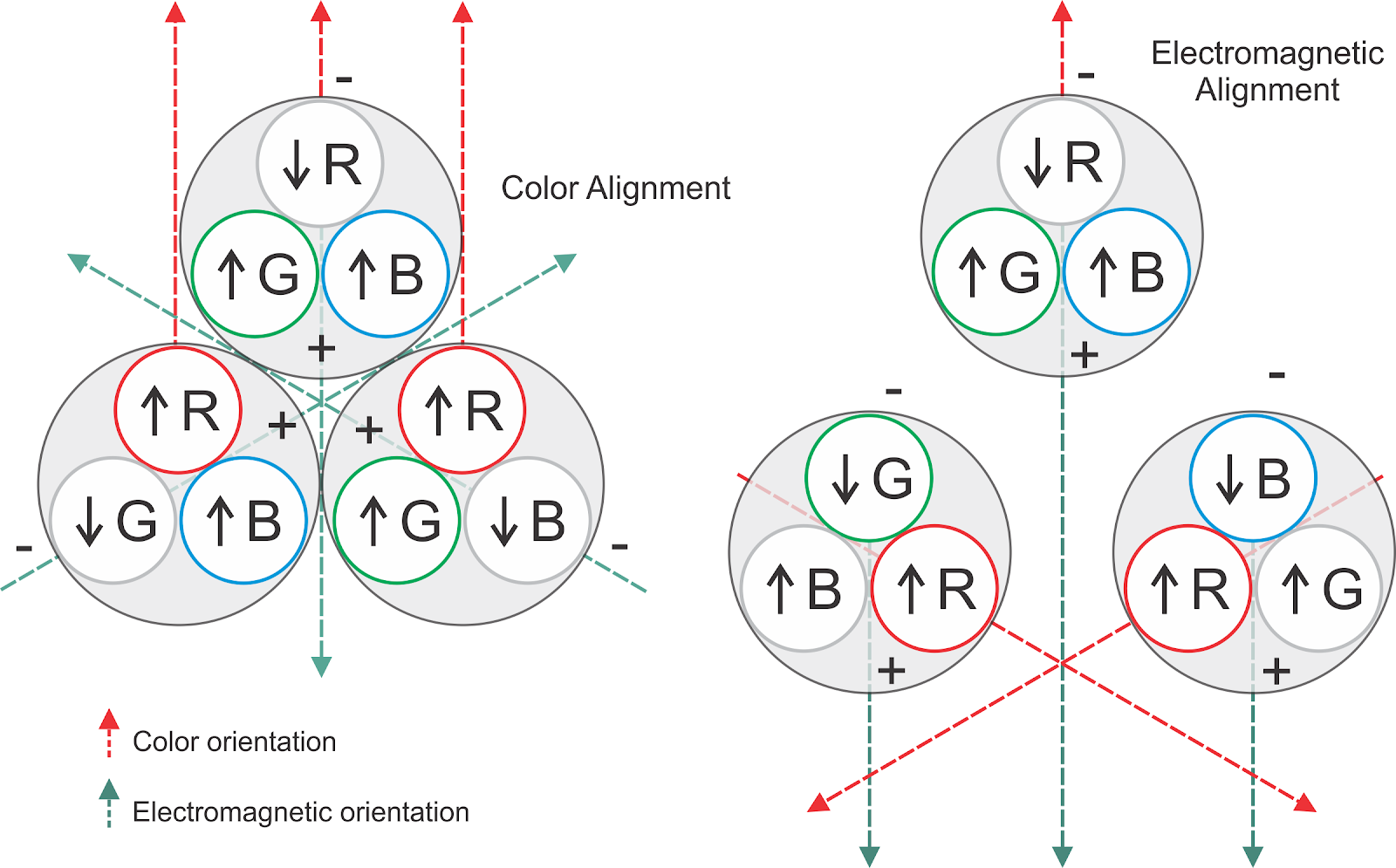Ignoring the specific properties of atoms, nuclei and their interactions for a moment, is there anything that can be derived from the general characteristics of spatial density that could help us understand the impacts of changing the density of a nucleus as shown above?

Copper has an atomic weight of 63.546 amu (atomic mass unit). It has 29 protons and 35 neutrons. The commonly accepted radius of its nucleus is 145pm (picometer). The nucleus of atoms can take many shapes depending on the arrangement of the nucleons within them, but if we assume for our purposes that they are spherical, we can begin to work through scenarios to prove an important concept related to density.

The currently accepted radius of a proton is about .87fm (femtometer). If the 64 nucleons of a copper atom were packed as tightly as possible (29 protons + 35 neutrons = 64), the radius of the nucleus would be 3.847fm. This is based on the Kepler Conjecture, which asserts that the maximal density of spheres (nucleons in our case) that can be packed into a larger sphere (the nucleus) is about 74%. This value is best case in the pristine terms of Euclidean Geometry, but will sufficiently serve our purposes. On the lower end, randomly arranged spheres can have a much lower volume of ~64%.

The distance of separation of nucleons that can be achieved through the alignment of color charges has yet to be calculated. Regardless of what the distance happens to be, calculating its impact on the overall density of the nucleus will be a trivial matter. If alignment of color charges is possible, and we were to assume for sake of argument that the nucleus can be dilated to a point where the resulting space between nucleons is equal to as little as half of their diameters (one radius), then the overall density of the nucleus will drop from 74% to 28%, as depicted in the image below.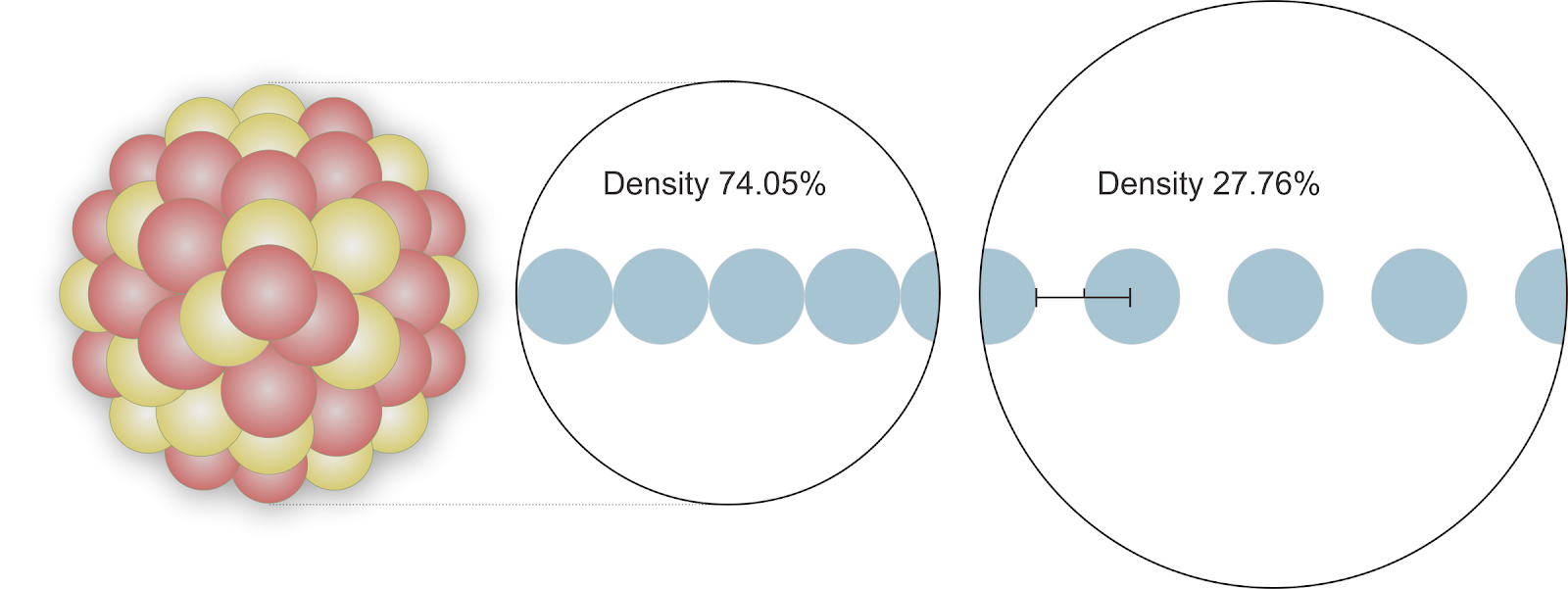Presuming that modifying the nuclei density of a grouping of copper atoms from 74% to 28% would have no impact on the radius of the atom (although there would likely be an impact to the shape of the electron orbital shells due to the restacking of nuclei), there would be no change to the amount of mass in a mole, for example. Meaning, a cube of copper would contain the same number of atoms, the same number of nucleons and the same number of quarks whether dilated or not. The mass would be the same, but the density would be markedly different.

Thinking of the gravitational attraction between the nucleons within the atom in terms of spacetime curvature, we could assert that the attraction between the nucleons would diminish by the square of the distance between them, as depicted below.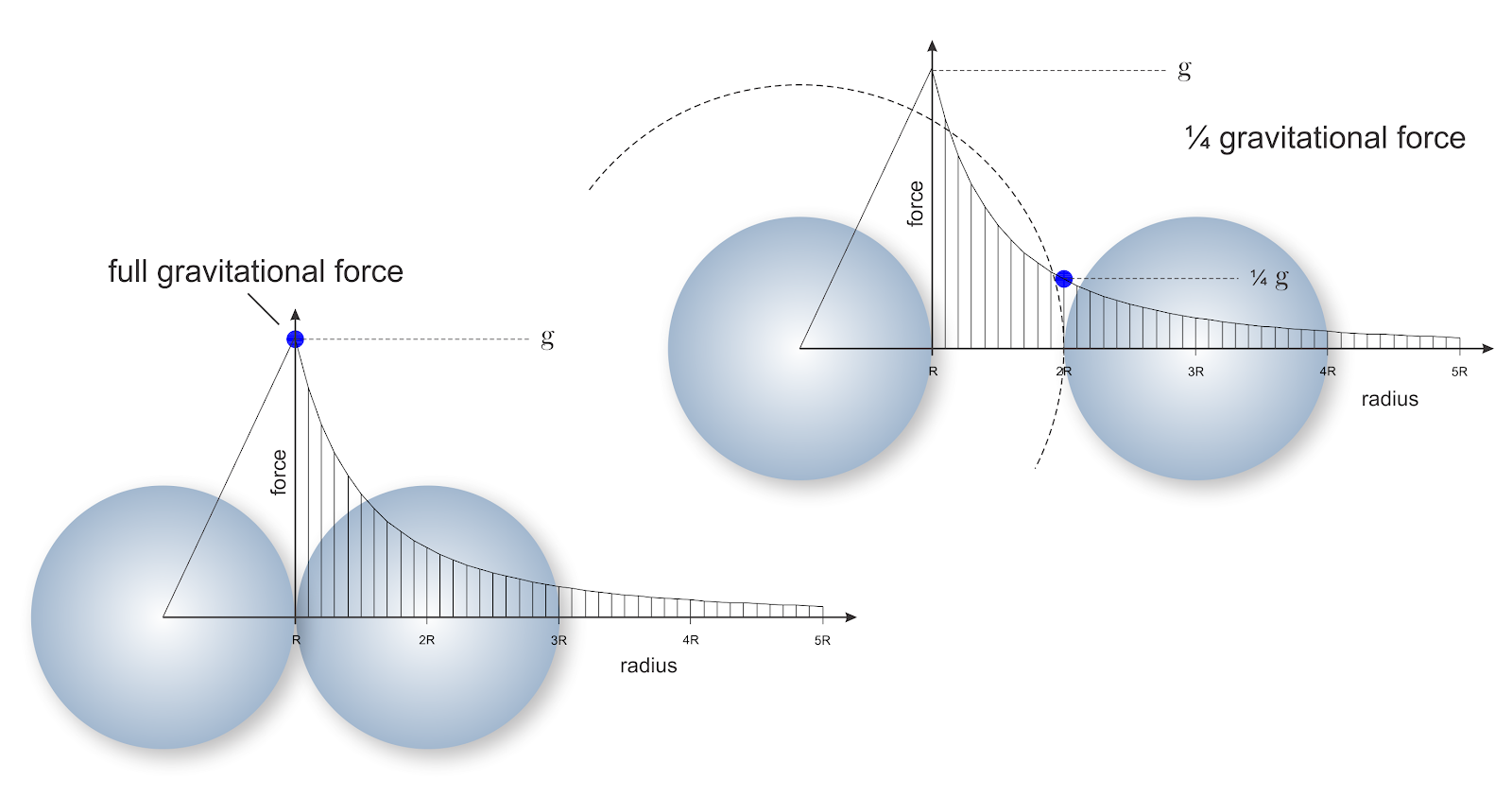As we know, the force of gravitational attraction is a function of spacetime curvature. The only explanation for the two protons on the upper right to experience ¼ the gravitational force as those on the lower left is less spacetime curvature.

When it comes to spacetime curvature, it is not the density of atoms that matter, per se; but the density of the nucleons within them. As outlined earlier, atoms are not indivisible units of matter. When groupings of atoms coalesce in sufficient quantities to produce appreciable spacetime curvature (gravity wells), the nucleons within those atoms are where material impact occurs. This means that although the overall mass of a given quantity of copper, for instance, would not change if their nuclei were dilated in this way; the distribution of mass within them would result in a shallower gravity well because the dilated nuclei would be defocused, resulting in less curvature, and therefore, less gravitational force. Specifically, if the nucleus of a copper atom were dilated as described above (the density of copper is 8.92 g/cm3), its density would drop to only slightly more than that of silicon (2.33 g/cm3).

In terms of gravitation, matter moves, and in fact, accelerates towards other matter due only to the overlap of the individual gravity wells that surround them. Whatever the scale and quantity, these are base behaviors. The Earth, Mars, the sun, and even an atom, produce gravity wells. They range in size and strength, but anywhere there is matter, there is a corresponding impact to the shape and curve of spacetime. If we imagine the collective gravity well produced by a group of atoms, and that the mass within those atoms is de-densified, the impact would be modification to the curvature of spacetime.

Many questions remain, such as:
• How strong will the electromagnetic field have to be to influence the orientation of protons? Given that the Strong Nuclear Force is 137 times stronger than the electromagnetic force, the value will express as a measure of rotational torque that must be applied to the proton.

• Given the extremely short range of the Strong Nuclear Force, the extent to which a nucleus can be dilated without breaking the nuclear bond between nucleons must be determined. What are the boundaries?

• Will nuclei dilation impact the shape of electron shells? Dilation could free the protons and neutrons enough to allow them to restack in ways that impact the electromagnetic fields they emit, thereby reshaping the electron shells.

• Quarks continuously fluctuate in color. Will it matter, or will the repulsive phenomenon that occurs between adjacent, like colors simply transfer seamlessly from one state to the next? Given that the nuclear bond between quarks is unbroken during this natural process, it may be reasonable to assume that changes in color will have no impact on the mechanisms discussed in this paper.
There is also the question of the role neutrons will play in these mechanisms. Being possessed of two down quarks and one up quark, the net electromagnetic charge of a neutron is 0e. However, the expectation is that the force of torque will be conveyed from protons to neutrons via the color force, similarly to how a car with only one driving wheel nonetheless causes all four wheels to turn simultaneously as they carry the car forward. Under normal conditions, it is impossible to detect which of the four wheels is supplying the force that causes the car to move. Likewise, the electromagnetic field used to cause the collective protons of the nucleus to rotate must be sufficiently powerful to rotate the neutrons as well, overcoming the resistance of the color force for themselves and the neutrons. This suggests that, in general, elements with lower proportions of neutrons in ratio to protons may be more efficient materials for antigravity.

Finally, nucleons are not arranged as neatly in reality as they have been depicted here. They are clusters of protons and neutrons, in which the alignment of color charges must play out in three dimensions. The efficiency of the systems discussed here will be a factor of how those nucleons are arranged.

Going forward we'll look at a quantity of light atoms compared with heavier, dilated atoms within a given area of space to get a better view of practical impacts.

The next question is the most obvious of all. How can this be done?